﻿ Accounting for the Geometric Factor in Classical GravityPublications are Open
Access in this journal
Article Versions
Export Article
• Normal Style
• MLA Style
• APA Style
• Chicago Style
Research Article
Open Access Peer-reviewed

### Accounting for the Geometric Factor in Classical Gravity

Alexey S Belyaev
Frontiers of Astronomy, Astrophysics and Cosmology. 2018, 4(1), 18-29. DOI: 10.12691/faac-4-1-2
Received September 10, 2018; Revised October 30, 2018; Accepted December 03, 2018

### Abstract

This paper shows that it is often necessary to consider the geometric factor establishing the relationship between the geometric dimensions of interacting objects and the distance between them when describing gravitational interactions within the framework of classical gravity. Especially when the size of an object is comparable to the distance to the reference point from the centre of its mass, including a spherically symmetric distribution of mass throughout the volume, it is unacceptable to replace it with a point mass located at the centre of mass of the object and is equal to the mass of the object. Another special case is the description of the gravitational field inside a material object with a mass distributed over its volume. For example, it is shown that within the spherically concentric cavity of a material object with a spherically symmetric mass distribution a gravitational field is present and that the substance inclusions in the cavity is gravitationally attracted to the wall of the cavity. One consequence of this conclusion is that the halo of large galaxies, consisting of a hidden mass, concentrates its material at the periphery, and does not form rarefied corona with a decreasing density of matter at the periphery.

### 1. Introduction

The nature of the gravitational interaction is still unknown. However, regardless of the essence of the forces of gravity, the laws of classical gravity must apply within the limits of the admissible domains of the accepted parameters for the gravitating system under consideration. In this case, classical gravity is based only on two positions: ’s law of universal gravitation and the principle of superposition.

Why gravitational interactions are described by the law of Newton-it is not yet known. However, this law is fulfilled with interactions traditionally related to classical gravity. Classical gravity considers systems whose objects are macro-objects, having moderate velocities relative to each other, and moderate mass densities.

The principle of superposition is when a complex gravitational influence on the object under study from objects of the gravitating system can be divided for an independent consideration of the gravitational interactions of each of the objects of the system with the object under study with subsequent vector summation of the resulting forces of these individual interactions. Currently there is no knowledge of the existence of screens of the gravitational field that restrict, weaken or amplify the field, nor about cases of interference or other inter-related influences on the test mass from several objects that have a mass.

When constructing the theory of classical gravity, the object, which is gravitationally acted upon by the system, is at first excluded from consideration for preliminary study of the gravitational field formed by the system. For this purpose, instead of an object, an elementary mass is taken, enclosed in an elementary volume, and alternately placed at all spatial points around the system to determine the resulting gravitational force acting on it. This is the so-called test mass. The characteristics of the gravitational field of the system are determined, through the obtained values of forces, which facilitate the subsequent description of its gravitational effect on the object.

All the individual forces of gravitational interaction of the test mass with the objects of the system and the resulting force of gravitational interaction with the system as a whole, are applied to the same geometric point in which the test mass is located (due to its elementality). However, all the reciprocal forces of gravitational interaction, acting from the test mass to the objects of the system, are applied to each object of the system separately and their application points are spaced out in space. If the objects are interconnected one way or another, not necessarily only due to gravitational interaction, then the reciprocal resultant force from the test mass to the associated system of objects is usually applied to the center of mass of the system. It is equal in modulus to the resulting gravitational force acting on the test mass from the system but is directed in the opposite direction. In fact, the system or simply an extended object, also a system, is replaced by a point mass equal to the total mass of the system.

In particular, the points are replaced by planets, stars and other cosmic objects that have spherical symmetry in the mass distribution. For example, for a double connected system whose objects are replaced by dots, equipotential surfaces of the effective potential are constructed while taking into account the centrifugal forces and the Roche lobe is defined, bound by the equipotential surface passing through the first point Lagrangian point. If the real geometric dimensions of one of the objects, taking into account its gas envelope, turn out to be larger or equal to the Roche lobe, it will become a source of flowing matter towards another object (Roche lobe is constructed with respect to real total masses of objects, but the objects themselves are contracted to points to which object masses are assigned).

However, it is important to be careful when performing such operations. First, it is necessary to take into account the distribution of mass inside the system, the external gravitational field of which is being studied, in the first place, pay attention to the presence of spherical (or another) symmetry in the distribution of mass. After all, the distribution of the mass depends on the distribution of the gravitational field around the system and the magnitude of its effect on the test mass placed at the control point. Secondly, the mutual gravitational attraction forces of a system with an asymmetric mass distribution and a trial mass located near the system do not lie on the line connecting the test mass with the center of mass of the system (the gravitational force from the trial mass tries to impart a rotational motion to the system).

For the case when the mass in a intertwined system has a spherically symmetrical distribution, the gravitational force acting on the test mass is always directed along the line connecting the test mass to the center of mass of the system and the test mass does not impact on the spin the system with its response. However, as will be seen from the material presented below, the reciprocal resultant force acting on the system from the test mass is not always applied to the center of mass of the system. The point of application of the response reaction that influences the system from the test mass is affected by the geometric factor, which depends on the ratio of the size of the system and the distance from the center of mass of the system to the test mass. In this case, the geometrical location of the point of application of the response determines the specific value of the calculated distance in the law of universal gravitation, which is squared. For this reason, the law of universal gravitation that is currently being used in fact seems to be distorted and, in certain situations, can lead to both inaccuracies and erroneous interpretations. For example, there are errors in the determination of Lagrange points.

In this case, the term "geometric factor" is introduced specifically for the gravitational interaction, in which gravitationally interacting objects have a spherically symmetric mass distribution, since in practice such objects are always (!) replaced by point masses, and the geometric factor is never (!) taken into account. The geometric factor is usually considered in the absence of spherical symmetry in the mass distribution. However, since for astronomical sciences these cases are quite rare, the term "geometric factor" is simply absent. It is more often used in the theory of the electromagnetic field.

But there is one more nuance that frequently introduces inaccuracies into the produced estimates of gravitational interactions. In modern astrophysics it is accepted that the motion of an object in a stationary gravitational orbit is affected only by the mass that is enclosed within the trajectory of its orbit if the mass of external objects is distributed centrally symmetrically relative to the center of mass of the system. Since any stationary orbit for a system with central symmetry is always periodic, then if it is not circular, it must take the form of an ellipse over time. Convergence of orbits of various objects of the system may disrupt their trajectories. It is also required to adjust for the actual distribution of the matter density. However, at the primary stages of construction of models, this can be neglected, and corrections can be introduced later in the process. In a circular motion, due to compulsory equality of the forces of gravity and centrifugal forces, each radius of the orbit has its own specific and uniform in absolute value linear velocity. In the elliptical orbit, the absolute value of velocity is variable, but the root-mean-square value of its radial component also uniquely characterizes the value of the major axis of the ellipse. It is assumed that when moving along the ellipse only the internal mass affects the root-mean-square value component of velocity along the semimajor axis, however, internal not for an elliptical orbit, but for a circular orbit, with a diameter equal to the major axis of the ellipse. Figure 1 presents the above said in a diagram form.

• Figure 1. Zones of the gravitating system influencing on the orbital motion of the object under consideration

When external masses do not exert gravitational influence on internal masses, a simple interpretation is adopted within the framework of classical gravity: the gravitational effects of external masses on the internal masses generally compensate each other.

This interpretation has arisen because of the existence of an alternative way of describing classical gravity in the language of vector analysis. Gauss theorem, which is among the basic provisions of the vector analysis, states that the flux of the field vector of a potential vector field along any closed surface surrounding a set of field sources is proportional to the total value of the characteristics of the sources inlying inside the surface (the sum of characteristics that uniquely determine the source of the field). For the gravitational field, the determining characteristic of the field source is mass.

A corollary of Gauss's theorem is that the gravitational field inside a spatial material object with a spherically symmetric mass distribution is determined only by the mass of the core extracted from the object by a concentric sphere passing through the point for which the potential of the gravitational field is determined. If there is emptiness inside the selected middle zone, then the gravitational forces do not act on the bordering elements that create the cavity. Moreover, the corollary of the Gauss theorem excludes the gravitational interaction of two foreign inclusions with a mass inside the cavity. These features of the corollary of the Gauss theorem allow theorists to replace the mass distributed with spherical symmetry in space by a point mass located at the center of symmetry and equal to the total mass, which is usually the case in all calculations.

The incorrectness of the position resulting from the corollary of Gauss's theorem about the absence of a gravitational field inside the concentric spherical cavity of an object with a spherically symmetric mass distribution was indicated in article 1 by Belyaev. Moreover that work, in the process of developing the basic scheme of spontaneous gravitational compression of dust clouds in outer space, demonstrates the inevitability of violating the corollary of the Gauss theorem. The absence of passivity of peripheral zones on process of gravitational compression leads to dust accumulating, during compression of a space clouds, in peripheral zones of a cloud. One specific result of this result is the conclusion that there exists a massive halo of the Universe. The same conclusion was made in an independent work by Belyaev 2 but with using different independent starting positions. The existence of a massive halo of the Universe sheds light on the nature of cosmic X-ray background radiation and on many other issues that will be discussed in other works. In the meantime, in the development of the topic of cosmology, in Belyaev 3 an approach was proposed for the development of a new model for the birth of the Universe.

The basics of the theory of classical gravity can be found, for example, in Misner et al 4. In general, classical gravity is considered so well-studied that it is impossible to create something fundamentally new within its framework. However, it was shown in Belyaev 5 that gas clouds contracting in outer space, if their compression initially originated by self-heating, cannot change their heating to cooling without qualitative changes (when the cloud ceases to be a gas cloud). Thus, the red and brown dwarfs should not be able to cool down, as is generally accepted today; that under the influence of gravity even very light clouds, for which it is considered that they are only able to dissipate, should be able to undergo compression. Another unexpected result obtained within the framework of classical gravity is the possibility of spontaneous gravitational compression of cosmic gas clouds with simultaneous cooling (see Belyaev 6). This article attempts to build a bridge to other new displays of classical gravity. Particularly, this paper will consider the structure of the halo of large galaxies consisting of hidden mass.

When a large part of the mass of the system is concentrated in the allocated spatial region, the parameters of the orbital motion of the satellites around this region are determined by the mass of the selected region. For example, the largest part of the solar system is concentrated in the Sun, so the velocity of the planets and the geometry of their orbits provide a reliable estimate for the mass of the Sun. Kepler described the planetary orbits of the solar system using the astronomical observations of Tycho Brahe. Kepler's empirical correlations allowed Newton to formulate the law of universal gravitation. For this reason, various orbits of different objects around the same dominant gravitational mass are called Keplerian orbits.

The resulting experience of estimating the dominant mass was subsequently used to determine the masses of galaxies. This allows the velocity curve of stars or gas clouds of a galaxy to be constructed. At the beginning, as the stars of the galaxy are increasingly distant from the center of mass, their velocities increase because the larger the orbit of the star, the greater the mass is enclosed within this orbit. This is quite an expected effect. It was assumed that at a certain distance from the center of mass of the galaxy, the velocity curve would bend and begin to depict the Keplerian orbits. At this point the velocities could be used to estimate the mass of the galaxy.

However, an unexpected result of the research is that most large galaxies have hidden mass, which does not allow the Keplerian orbit section to appear on the velocity curve. The conclusion about the presence of a hidden mass was obtained in various ways, and it can be considered indisputable. Nevertheless, after establishing the fact of the presence of hidden mass, it is still necessary to determine the spatial distribution of this mass, regardless of the nature of the hidden mass and its kind (even if it is taken as dark matter). Today, such a description is made using the above-mentioned principle of non-influence of external masses.

However, this principle, which is a result of the corollary of Gauss's theorem, contradicts the existing theories of gravity. This point has not been considered by anyone up to now. Quantum gravity and the general theory of relativity deny the annihilation of the gravitational field inside the cavity of the material body. It is impossible for virtual gravitons or other carriers of the quantum field in the framework of quantum gravity, to simply disappear inside the cavity, however, within the framework of the general theory of relativity, according to its basic positions inside the cavity space-time is bent, with spherical equipotential surfaces which are denser closer to the walls of the cavity, that is, the potential inside the spherical cavity will not be the same for any point of the cavity. Therefore, the goal of this paper is to determine how, in this situation, which, in its essence, also relates to the influence of a geometric factor, the laws of classical gravity work within the classical interpretation, and not in the vector analysis interpretation.

### 2. Main Aim

The problems mentioned above, connected with the corollary of the Gauss theorem, require correction of the positions of the vector analysis. However, the positions of vector analysis are considered to be so flawless that before making the intended step towards their correction it is desirable to demonstrate the discrepancy between the conclusions of the corollary of the Gauss theorem and the truly classical interpretation of gravity on the basis of the law of universal gravitation.

The aim of this paper is to evaluate the applicability of the law of universal gravitation for various geometric relationships between gravitationally interacting objects with a spherically symmetric mass distribution. To this end, the distribution of the gravitational field near and within extended objects having a spherically symmetrically distributed mass will be studied. This analysis will make it possible to better understand the nature of the gravitational forces and will give an additional criterion for creating a final version of the theory of gravitation in the future. The basic axioms on which the analysis is based are the universality of the principle of superposition and the rigidity of its implementation in those cases for which it is customary to use the theory of classical gravity (supermassive objects, relativistic velocities and gravitational interactions of elementary particles will not be considered). The principle of superposition implies that the gravitational fields of individual elements of the system are always reliably described through their impact on the test mass, which follows Newton’s law of universal gravitation. The question of whether Newton’s law is fulfilled for the entire system, if it is replaced by a point mass, is the subject of analysis.

This paper only highlights the problems within the theory of gravitation. The calculations below should not be taken as a mathematical justification. They serve only to illustrate and to identify trends in the distribution of the gravitational field when describing it within the framework of classical gravity.

### 3. The Influence of Geometric Factors on the Law of Universal Gravitation

Within the framework of vector analysis, it is possible to determine the lines of force of a potential field of a set of field sources. For example, the gravitational field of a dipole consisting of two identical masses will differ from the gravitational field of a point mass with a double mass. Another formulation of the problem is also possible. For example, the identification of the best approximation to describe the gravitational field of the aggregate of gravitational masses. After all, the aggregate mass can be replaced not only by a point mass, but by a dipole, a quadrupole, and other configurations.

The purpose of this paper is to evaluate the effect of an object extended in space with a spherically symmetric distributed mass on the test mass within the framework of classical gravity. To do this, it is necessary to record the impact of each material point of the object in the form of the law of universal gravitation on the test mass, and then vectorially add up all the results obtained. Since a circle, a sphere and even a ball with a uniformly distributed mass can be obtained not from a collection of material points, but from a set of dipoles located in parallel, it is first necessary to estimate the gravitational effect on the test mass of individual dipoles that make up a spherically symmetric object, followed by an estimate of their total impact on the trial mass.

Consider the result of the gravitational influence of two identical masses m on the test mass dm. Let the masses m be placed on a circle of radius r symmetrical to the straight line connecting the test mass dm with the center O of this circle (see Figure 2). The distance from the mass dm to the center O will be denoted by R. Let us consider the gravitational interaction with the mass pairs m' and m" at any arbitrary symmetric points 3' and 3'' of the circle. Points 2' and 2'' correspond to the maximum of the varying angle α for different pairs under consideration with masses m (m' = m and m" = m) for each specific distance R. We define the vectors of forces acting on the mass dm as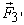and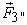according to the principle of superposition and add them vectorially.

• Figure 2. Diagram of the gravitational field of a spherical object

First, let us consider the pair of 1'-1'' lying on the line joining the test mass dm to the point O (the masses m' = m and m" = m located at points 1' and 1", respectively). The modulus of the total force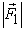for a specific case (α = 0, cosα = 1) is equal to the simple sum of the moduli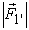and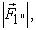and the direction coincides with the direction towards point O. If the constant Gdm in the universal gravity formula, where G is the gravitational constant, is denoted by b (b = Gdm) then the gravitational force acting on the test mass from the pair of masses m' and m'' located at points 1' and 1'' will be equal to: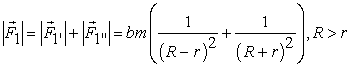(1)

Taking into account that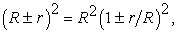we get: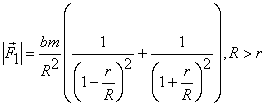(2)

For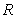>>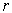the second terms in the denominators of formula (2) tend towards zero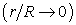, therefore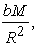where M = m'+ m'' = 2m, i.e., the law of universal gravitation for the total equivalent point mass placed at the center of mass of two objects is satisfied. However, as r increases with respect to the distance R, the principle of replacing the system by a point mass located at the center of mass and equal to the sum of these masses becomes inadmissible, since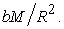Moreover, for Rr (or for rR) we get→ ∞. This means that the gravitational interaction, characterized by an inverse quadratic dependence on the distance, cannot be linearly added up from individual parts of the object: the closer parts of the object begin to significantly dominate in terms of their influence on the test mass in comparison with the parts located further away. This is a well-known fact, nevertheless, it requires special attention: the gravitational effect from the elements of the object close to the test mass is so strong that it is difficult to expect to replace the spherically symmetric object by a point mass with the total mass of the object at the center of, rather than near the object.

For clarity, this effect is demonstrated in Table 1 with the assumption that the shoulder of the gravitational dipole deployed parallel to the direction to the test mass is constant: r = const, and the R is the variable. Even at a distance 100 times greater than the shoulder of the gravitational dipole (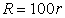), the replacement of dipole by a point mass located in the center of mass of the dipole is satisfied with an accuracy of only 1% (the dipole is deployed parallel to the line connecting its center of mass with the test mass).

Let us continue our consideration of the effects of double masses on the test mass. In a general case of two masses m located at points 3' and 3'', with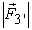=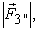the vector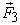forms a diagonal of a rhombus with length: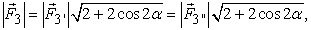and the direction of the vector coincides with the direction towards point O. Taking into account that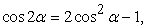we get the following (R > 0, the distance is calculated from the location of the test mass dm to point O):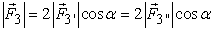(3)

Since we are considering pairs of objects m' and m" to be symmetric with respect to the line connecting their center of mass with the center of mass of test mass, and the objects themselves have the same masses and sizes, the distance from the test mass dm to the center of mass of two masses m is equal to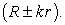The distance from test mass dm to masses m' and m'' is equal to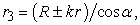where k is a fraction of r characterizing the deviation of the center of mass of two objects from the center O, with R > r (see Figure 2), therefore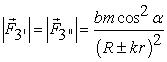(4)

Taking equation (3) into account, we get: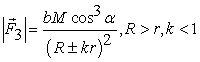(5)

With>>we get: cosα → 1. Therefore, according to equation (5), the gravitational field of a system of two objects at large distances from the system in comparison with its geometric dimensions is equivalent to the gravitational field of a point mass with mass M = 2m located at the center of mass of the system (the distance from the test mass to the center of gravity of the gravitational dipole is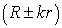). At the same time, the intensities and potentials of the gravitational field of the gravitational dipole coincide with the intensities and potentials of the gravitational field of an equivalent point mass, for>>the law of classical gravity, in the case of replacement of a dipole by a point mass, is satisfied.

However, as r increases (or R decreases), a geometric factor enters into effect, and the additivity of the dependence of the universal gravitation is violated: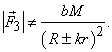This means that near the gravitational dipole the law of universal gravitation, allowing the replacement of a dipole by an equivalent point mass, placed at its center of mass, does not work. Whether to reduce the equivalent point mass or to move its placement away from the actual center of mass-should be decided for each specific case independently, depending on the goals being set.

Before continuing with the generalization of the gravitational effect on the test mass not from pairs of gravitating objects, but from a gravitating sphere made up of a set of similar pairs, let us also consider the effect on the test mass of the pair 2'-2". The distances to the objects m' and m" located at points 2' and 2" are equal to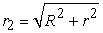regardless of the relation between R and r (R > 0, i.e., R can have any value, it could even be less than r). Thus,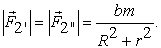Hence, taking into account equation (3):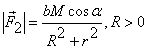(6)

In this case, a trend observed in the variation of the gravitational field along the central perpendicular to the axis of the dipole appears more clearly than in the more general case when pairs 3'-3" are considered. The closer the values of R and r are to each other, the more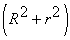/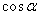deviates from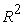(for the expected classical principle to be fulfilled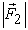= bM / R2), and the more the replacement of the gravitational dipole by the point mass located at the center of the dipole mass becomes unacceptable. When the test mass closely approached the sphere on which the pair 2'-2" is located, i.e., when the masses m have the same distance from their common center of mass as the test mass dm from its center of mass (R = r, α = 45), the modulus of the resultant force on test mass is equal to: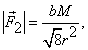i.e., approximately 3 times weaker than in the case with an equivalent mass at the center of mass of the pair. With convergence of the test mass to the pair 2'-2" which is already inside the circle (R = kr), the angle α continues to increase. At α = 60, the distance from the test mass dm to the center of the circle O is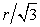(k ≈ 0.58), and the modulus of the resultant force to the center of mass of the pair 2'-2" is equal to: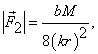i.e., will decrease in comparison to the system with a point equivalent mass located in the center of mass of the pair, not three, but 8 times. With further convergence of the test mass to the center of mass of the pair 2'-2" the resulting force acting on it will continue to decrease down to zero. The presented regularity is a clear demonstration of the impossibility of placing an equivalent point mass with mass M at the center of the dipole mass when the trial mass is close to the dipole. But the dipole does not have spherical symmetry, so there is nothing fundamentally new yet.

To proceed let us consider the gravitational field of the set of dipoles that make up objects with spherical symmetry. First, let us consider a flat surface whose plane coincides with the plane of the Figure. Since the dipoles 3'-3'' are symmetrical and perpendicular to the straight line (1';1'') passing through the test mass and the center of the circle, the vertical components of the forces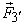and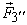for each dipole mutually compensate each other, and the sum of the horizontal projections determines the attraction force of the dipole to a trial mass dm, which can be applied to an equivalent point mass located at the center of the dipole mass on the straight line (1';1").

To estimate the possibility of replacing a circle with a uniformly distributed mass along its length by a point mass located at the point O and equal to the mass of all the dipoles that make up the circle, it is necessary to estimate which semicircle, left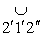or right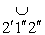, will be more attracted to the test mass dm. It is obvious that the equivalent point masses of semicircles will be located on the segment [0;1'] to the left of point O for the left semicircle and on the segment [0;1''] to the right of point O for the right semicircle, to which the sums of the horizontal projections of forcesandof all dipoles forming semicircles will be applied.

In order to replace the circle by a point mass with the mass of two semicircles and with location at the point O, it is necessary that the forces of their attraction to the test mass dm are the same. It is difficult to make such an estimate using formula (5), a more rigorous approach is necessary. The uncertainty in the estimation when using formula (5) is made by the parameter cosα, which in its physical essence determines the values of the horizontal projections of forces,. However, even at this stage there is sufficient information to make an estimate without using formula (5). Since the masses of the semicircles are the same, and their equivalent point masses lie on the straight line (1';1") on opposite sides of the point O, the force of attraction of the left semicircle to the test mass is inevitably greater than the attraction of the right semicircle to the test mass. This means that the equivalent point mass of the circle, if its mass is equal to the mass of the circle, will be located not at point O, but to the left of it. This is a very important and undeniable conclusion.

It is obvious that the result cannot change the transition from a flat to a three-dimensional space: the left hemisphere will be attracted to the test mass more strongly than the right hemisphere, and the location of an equivalent point mass whose mass is equal to the mass of the sphere will inevitably shift from the central point O to the trial mass along the straight line connecting the point O with the test mass.

If we consider multiple spheres simultaneously, then same conclusion can be obtained for the ball: its left hemisphere will more strongly influence the closely located test mass in comparison with the right hemisphere. Furthermore, the direction of the vector of the gravitational force created by the ball and acting on the test mass, due to the axial symmetry, is directed toward the center of mass of the system. However, the modulus of this force will be greater than what it should be according to the law of universal gravitation, when the ball is replaced by an equivalent point mass, has the mass of the ball and is located in the center of the sphere. This case is in the first approximation equivalent to the case with a dipole unfolded along the line of sight (for the pair 1'-1'') as shown above. Accordingly, if the mass of the ball is assumed to be strictly prescribed beforehand, then the location of an equivalent point mass equal to the mass of the ball will be closer to the test mass than the center of mass of the ball.

The set of spheres that make up the model ball can have spheres composed of dipoles of different masses (but the mass of the dipoles for each particular sphere must be the same). The density of the repetition of the concentric spheres that make up the ball can also be different. If the ball is assumed to be inhomogeneous with increasing density towards its center (when either the masses of the objects mi or the density of these objects with mass m increase), then the deviations from the law of universal gravitation, when the ball is replaced by an equivalent point mass with a mass equal to the mass of the ball, will have the same character (regardless of the level of density variation allowed within the framework of classical gravity), but the inaccuracies of the operation of replacing the ball with a point mass located at its center of mass will decrease.

The replacement of extended objects with a spherically symmetric mass distribution by an equivalent point mass located at the center of the object and having the same mass is common in astronomical sciences, which leads to a multitude of arising inaccuracies. In reality, it is only possible to make such a substitution when taking into account the geometric factor. Currently the substitution is admissible without taking into account the geometric factor due to the existence of the corollary of Gauss's theorem, an error, which will have to be demonstrated in the near future. It is possible that this will be achieved by the readers, or the author, in the near future.

This can be illustrated by Earth’s gravitation. Close to the surface of the Earth, bodies are not attracted to the center of the Earth, but only in the direction of the center of the Earth. Moreover, the gravitational influence of the Earth cannot be replaced by the influence of an equivalent point mass located at the center of the Earth's mass and equal to the mass of the Earth. Such operations are only permissible when the motion of the Earth as a satellite of the Sun is being considered. For objects gravitationally attracted to Earth's surface, it will be necessary either to reduce the distance to the location of the equivalent point mass along the line, going towards the observer from the center of the Earth, or to increase the value of the effective mass of the Earth.

One can argue that there currently exists a fairly successful applied science which gives real practical results-gravimetry. Regardless of the set aims, gravimetry sets a reference field of gravity for the entire surface of the Earth at sea level. This is the so-called normal field of gravity, the dependence for which is derived semi-empirically. First, theoretical dependencies for the gravitational potential and centrifugal forces are determined (the centrifugal forces are inversely proportional to the distance from the rotation axis). The model determines the gravitational potential on the surface of the earth by assuming a layered uniform distribution of the density of matter within the Earth. If there are layers with a different density, they are detected during measurements and are mapped. To solve new problems of gravimetry the results obtained earlier through carrying out new measurements, are taken into account in a summative gravimetric chart which contains the values of the normal gravitational field. When compiling this map, the normal gravitational field is also corrected for the deviations of the Earth's shape from the ellipsoid of rotation. Later, corrections are made to the height of the measurement, to the influence of the masses between the measuring point and the sea level, on the location of the Moon and the Sun in all ongoing gravimetric studies. Corrections are carried out even on the relief of the surrounding terrain around the measuring point (the average height of the relief above sea level is important) and weather conditions (the mass of the "outside" air, in contrast to a similar cosmological scale problem when studying the corona of galaxies, is considered an influencing factor). As a result of gravimetric research, the so-called anomalies, which are the deviations of current measurements from normal gravity, are found. The anomalies found allow one to make a conclusion about the problem being currently solved.

At first glance, it seems that the existing applied aspects of the classical theory of gravity already take everything into account. However, the accuracy of the obtained results, in this case, is explained only by two factors. First, the density of the Earth is not uniform and grows towards the center. Therefore, the accuracy of the approximation, allowing the use of the law of universal gravitation with an equivalent point mass, located at the center of mass of the Earth, increases. Secondly, all the other factors which influence the magnitude of gravity are quite significant, so empirical approximations need to be made, which, smooth out all the theoretical inaccuracies.

It is also useful to pay attention to the errors that arise when determining Lagrangian points for pairs of cosmic objects whose geometric dimensions are 1% or more of the distance between them. For example, the size of the Moon is about 0.5%, and the Earth's size is about 1.7% of the average distance between the Moon and the Earth. The error in determining the zones of Lagrangian collinear points will be the most sensitive for this situation.

The main conclusion of this section is that when determining the gravitational potential around an object, including an object in which the mass is distributed with spherically symmetry, it can be replaced by a point mass located in the center of mass of the object only when the distance from the object substantially exceeds the geometric size of the object.

### 4. Gravitational Field inside Extended Objects

From the arguments presented above, it becomes clear that the corollary of Gauss's theorem appearing in the description of classical gravity in the language of vector analysis contradicts the theory of classical gravity described by the law of universal gravitation in combination with the principle of superposition: the gravitational fields inside the concentric spherical cavity of a material object with a spherically symmetric mass distribution exist and affect on the test mass.

Consider the test mass dm inside a circle consisting of pairs of objects mi' and mi" of the same mass (mi' = m and mi" = m), which are symmetric, as before, with respect to the straight line connecting the test mass dm with the center of the circle O (see Figure 3). Let us change the notation of distances a little, with respect to the notation used previously. Let R denote the radius of the circle, the small r is the distance from the test mass dm to the arc, supported by an arbitrarily chosen symmetrically arranged pair of masses at the points 3' and 3", kr is the distance from the test mass dm to the center of mass of the pair (k < 1). The points 4' and 4" correspond to the case when the center of mass of the pair under consideration coincides with the location of the test mass (k = 0). Points 5' and 5" characterize the right pairs of objects (relative to the test mass) of the left semicircle. The points 6' and 6" refer to the pairs of the right semicircle, when pairs of masses considered are outside the semicircle in which the test mass is located. Points 2' and 2" characterize the joint of two semicircles, for which the test mass from the inner semicircle for one of the semicircles becomes external for the other semicircle. The points 1' and 1" are the vertices of the arcs that are supported by the pairs under consideration.

• Figure 3. Diagram of studying the gravitational field inside a spherical object

For the pair 1'-1'' it is obvious that the test mass will be more effectively attracted to the mass m' = m, the distance to which is r than to the mass m" = m, the distance to which is (2R-r).

For pairs 3'-3'' it is obvious that the law of universal gravitation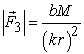(in the case of replacement of dipoles by an equivalent point mass with mass M and its location at the center of the dipole mass) will be violated: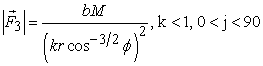(7)

(formula (7) is obtained by analogy with formula (5)).

As angle φ increases, the gravitational effect of the pair on the test mass gradually weakens because the forces,are increasingly compensating each other. The pair 4'-4'', with φ = 90, completely stops having any gravitational effects on the test mass. This tendency leads to a violation of the principle of replacing the dipole by a point mass located at its center of mass. If we would gradually decrease the angle φ from 90 the modulus of the force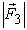will growth with the simultaneous growth of the distance kr from the test mass to the center of mass of the pair 3'-3", which must be substituted into the universal gravity law formula for the gravitational dipoles with constant mass. In this case, the growth of the resulting gravitational force acting on the test mass, despite the divergence of the center of mass of the pair from the test mass, occurs, firstly, by reducing the distances from the test mass to the points 3' and 3" moving along the arc of the circle in the direction to the point 1' (i.e., due to the growth of forces,), and, secondly, due to the increase in the projection of forces on the direction to the center of mass as the angle φ decreases. At the same time, the number of pairs under consideration increases with each following unit of angle φ (for a uniform distribution of objects with masses m along the circle, their quantitative density depends on the angle φ).

Having said that, for planar case we can conclude that if the test mass is placed in the left semicircle at the center of mass of the pair 4'-4", then the gravitational action on it by the left arc, supported by the chord [4'; 4''], the main contribution will be made by the elements at the top of the arc. Therefore, in the first approximation, we can assume that the arc cut off by points 4' and 4'', with length 2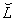and linear density ρl, with mass 2ρl, can be replaced by a point with the same mass (point mass) by placing it at the peak of the arc (at the point 1'). The actual distance to the point to which to apply when replacing the system by an equivalent point mass, the reciprocal gravitational force from the test mass, will be slightly less. This means that in our estimates, we somewhat underestimate the gravitational force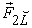acting on the test mass from the left arc, which will be directed towards point 1', and its modulus will be equal to: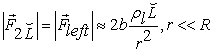(8)

The direction of the opposing force from the right arc will turn out to be strictly opposite. When considering pairs 5'-5'', which are increasingly approaching points 2' and 2'', the resulting gravitational dipole force will start to increase from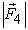= 0 to the maximum fracture value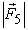. Therefore, the geometric point chosen for the replacement of two arcs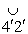and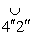by an equivalent point mass will be located, in comparison with the real center of mass of these two arcs, closer to the point O. In the case of pairs 6'-6", already located on the right semicircle, the resultant forces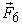will begin to weaken as they approach the location of pairs to point 1". However, this decrease will occur against the background of the growth of the projection of the force on the straight line (1'; 1'') and the growth in the number of pairs under consideration per unit of angle φ. Therefore, the geometric point for the replacement of two arcs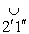and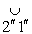of the point mass will be located, in comparison with the real center of mass of these two arcs, closer to the point 1" (further away from the test mass). It follows that in evaluative calculations the geometric point for the replacement of two combined arcs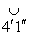and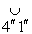, in the case of r << R, can be located at a distance R from the test mass dm, located at the center of mass of the pair 4'-4'' of the left semicircle (real distance to the required point of substitution of an equivalent point mass will be larger, that is, this choice of distance will lead to overestimated values of the resulting gravitational force for the right part of the circle, and therefore, at the estimation stage, as required, the location of the point of application of forces will be selected with a margin). Taking into account that for r << Rl, l =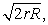we get: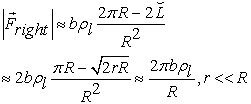(9)

Comparing expressions (8) and (9), it becomes evident that for r << R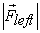>>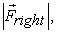that us to say, the test mass will be attracted to the wall of the spherical cavity with a force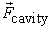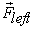the modulus of which, taking into account expression (8) and equation l =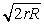, will be proportional to the distance from the test mass to the wall to the power of -3/2:(10)

All the reasonings provided are estimates. However, the law of proportionality between the gravitational force of attraction of the test mass to the wall of a flat cavity in the adjacent layers and the distance from the test mass to the wall of the cavity raised to the power of γ (in this case γ=-3/2) reflects a very real pattern.

All the arguments provided can easily be extended to a sphere. Further, if the thickness of the sphere turns out to be finite, then all the results first obtained for the circle, and then for the sphere, will also extend to the spherical layer. This means that near the wall of the concentric cavity of the centrally symmetric object, the force of the gravitational pull of the test mass towards the wall of the cavity will be proportional to the distance to the wall raised to the power of -3/2.

The generalized conclusion is the following: the test mass will gravitationally be attracted to the surface of the inner cavity of the voluminous object more actively the closer it is to the wall of the cavity. The degree of activity will be determined by a specially derived expression for the gravitational potential inside the cavity. Today it is believed that the test mass inside the cavity is in the state of weightlessness for any of its points. If we consider an object with spherically symmetrical distribution of mass and a concentric spherical cavity at the center, then, unlike the position adopted today, the test mass will not be attracted to the walls of the cavity of the object only at the central point of the cavity.

Thus, in the cavity of a massive body the gravitational field is present, and the gravitational potential grows in modulus as the closer it gets to the wall of the cavity. As a result, the adopted assumption that the gravitational potential inside a spherically symmetric object (without a cavity) is determined only by the mass enclosed within the concentric sphere passing through the point for which the potential is determined does not hold.

This conclusion can be further generalized as follows: the gravitational potential inside a massive object is not determined solely by the mass enclosed within a closed equipotential surface. The gravitational potential of an arbitrary point inside a massive body is affected not only by the internal mass of the object under consideration, but also by the external mass of this object. Moreover, when determining the gravitational potential from the internal mass, it cannot be replaced by an equivalent point mass located at the center of mass of the inner zone.

Thus, in fact, there are two opposing tendencies: on the one hand, the inner mass affects the considered test mass inside the material object more actively than it is accepted today in classical gravity, but on the other hand, the external mass relative to the test mass exerts a counteraction on the internal mass. Whether the resultant gravitational field inside the material object will be distributed, as today's classical gravity describes is the subject to further research.

### 5. Distribution of Hidden Mass in the Galaxy

So, most large galaxies have a halo consisting of a substance invisible to electromagnetic radiation. Today it is assumed that the halo of galaxies is a sparse extended corona. A similar conclusion is made for the following reason.

The square of the velocity of a satellite, given the circular orbit around the center of mass of gravitationally interacting objects, is proportional to the mass enclosed in its orbit, and inversely proportional to the radius of the orbit. This follows from the condition of equality of oppositely directed centrifugal forces and gravitational forces acting on the satellite. Therefore, the rotation speed of objects on Keplerian orbits should decrease inversely proportional to the growth of the square root of the radius of the orbit. However, observations show an approximately constant speed of motion of various objects outside the visible zone of the galaxy, for most large galaxies (even small satellite galaxies can be objects). A weak change in the fixed velocity is observed at distances of up to ten radii of the visible zone of the galaxy, after this point the fixed velocities decrease sharply.

If we assume that objects in the zone of a slight change of velocity are objects associated with the galaxy, i.e., perform finite motion, then, according to today's ideas, the observed velocities should be orbital velocities. Then, in accordance with the accepted principle of non-influence of external masses, the proportional growth of the internal mass enclosed in a volume bounded by a concentric sphere drawn through the location of the object should occur in the considered zone with increasing radius of the orbit. Therefore, firstly, the invisible substance is present in one form or another in all layers of the galaxy, and secondly, that the average density of this substance decreases layer by layer inversely proportionally to growth of the square of the radius of the layers under consideration. A substance can have both a continuous distribution in space and form lumps that do not have a high temperature. Hypothetically even clusters of dim stars are possible. The main thing is that the average density of any kind of substance should decrease according to the specified law.

However, as follows from the material presented above, the assumption about a tenuous corona of large galaxies with a layered uniform distribution of matter is erroneous. The peripheral layers are also influential in their gravitational action on the substance located between the central zone and the periphery. Therefore, the area around the visible part of the galaxies, as it follows from observations, is not only very rarefied, but almost completely devoid of hidden mass. Otherwise, the effects of inhibition of individual satellite objects in this zone would inevitably have manifested themselves in one form or another. This rarefied zone begins with distances measured from the center of the galaxy, gravitational forces of the inner and peripheral zones of which roughly balance each other out, i.e., from the adjacent outer layers to the visible region of galaxies that have approximately the same gravitational potential in depth of their thickness. The further away from the visible zone of a large galaxy to the periphery, the more rarefied space becomes, since in this zone of orbital motion no longer exists, but there is a substance "falling" on the periphery. It is only at the very periphery of the galaxy that the density of matter will increase sharply. For this reason, the halo of large galaxies is in fact a halo, not a rarefied corona with a continuously decreasing density of matter along its thickness, down to the peripheral region, indicating its outer boundary of which is rather difficult. The substance of the corona is concentrated exactly on the periphery, and therefore the word "halo" is applicable to it. If the radius of the halo exceeds the radius of the visible zone of the galaxy by about 10 times, then the halo mass should be more than 10 times the mass of the visible part of the galaxy.

Thus, the halo of the galaxy seems to almost form a second object of a bound pair system, in which the first object is the visible part of the galaxy. Such a corelated pair has two features. First, the objects of the system do not experience any orbital motion around the common center of mass. However, the substance between the objects can have an orbital motion. Secondly, it turns out that the first and second Lagrangian points are deployed into closed surfaces, and the third, fourth and fifth points are completely absent. The first Lagrange surface is the boundary of the visible part of the galaxy. The substance which is between the objects is distributed between them. If orbital motion takes place around the center of the galaxy in the Roche region relating to the first object, then in the Roche region related to the second object, the orbital motion can be associated only with the mandatory intersection crossing of this second object, i.e. only with periodic passes through the halo of the galaxy. This means that satellite galaxies of a large galaxy are constantly feeding the halo of a large galaxy. The origins of the halo of the large galaxy is a topic for further discussion connected with the analysis of a related stage in evolution of the Universe. Possible mechanisms for the formation of a hidden mass have been preliminarily indicated in 5, 6.

### 6. Conclusion

This paper demonstrates the incorrectness of the corollary of Gauss theorem. For example, when determining the intensity of a gravitational field outside an object that has spherical symmetry in the mass distribution, if the distances from the object to the control point are small compared to its diameter, it is inadmissible that the object be replaced by an equivalent point mass located at the center of the mass of the object and equal to its mass. Another example is the presence of a gravitational field inside the concentric spherical cavity of an extended object with a spherically symmetric mass distribution, which negates the corollary of Gauss's theorem, but confirms the general theory of relativity and the theory of the quantum gravitational field, as well as the results of this work performed within the framework of traditional classical gravity.

As a result, the gravitational effect of Earth on objects near its surface cannot be replaced by the action of an equivalent point mass located at the center of the Earth's mass and equal to the mass of the Earth. In this case, it is necessary either to reduce the distance to the location of the equivalent point mass along the line going towards the observer from the center of the Earth, or to increase the value of the effective mass of Earth.

Furthermore, due to the replacement of objects by point masses located at the center of mass of these objects, errors arise when determining Lagrange points for pairs of gravitationally interacting cosmic objects if their dimensions are not negligible in comparison with the distance between their centers of mass. The error in determining the collinear Lagrange points of the pair "Earth-Moon", although it will be less than 1%, will nevertheless be palpable.

The gravitational field inside the object is determined by both the internal and external relatively closed equipotential surface of the object's mass. Moreover, when determining the effect of the internal mass, it is not permissible to replace it with a point mass placed at the center of the masses of the inner part.

A new look at the distribution of the gravitational field near an extended object and inside it makes it possible to explain the reason for finding the hidden mass at a large distance from the visible part of the galaxy. Since the external mass of a system with a spatially distributed mass exerts an active influence on material objects within the system, large galaxies do not have a sparse corona with continuously decreasing density of matter along the thickness of the corona, but rather dense peripheral zones forming a halo of the galaxy consisting of hidden mass. Gravitational force near the spherical cavity, and, especially near the inner zone of the halo of the galaxy, is approximately inversely proportional to the square root of the cubed distance from test mass to the cavity wall (up to the halo of the galaxy).

### References

  Belyaev, A.S.: Gravitational Contraction of a Dust Cloud in Space. Universal Journal of Physics and Application, 10, 1, 22-30, (2016), http://www.hrpub.org/download/20160331/UJPA5-18406009.pdf. In article View Article  Belyaev, A.S.: Photon gas. Universal Journal of Physics and Application, 10, 1, 11-15, (2016), http://www.hrpub.org/download/20160229/UJPA3-18406008.pdf. In article View Article  Belyaev, A.S.: A new model of the birth of the Universe. Universal Journal of Physics and Application, 11, 5, 182-189, (2017), http://www.hrpub.org/download/20170930/UJPA7-18410201.pdf. In article View Article  Misner, C.W., Thorne, K.S., Wheeler, J.A.: Gravitation. W.H. Freeman and Company, San Francisco (1973). In article  Belyaev, A.S.: Trends of stellar evolution in modern physics. Universal Journal of Physics and Application, 11, 6, 247-263, (2017), http://www.hrpub.org/download/20171230/UJPA5-18410784.pdf. In article View Article  Belyaev, A.S.: The Possibility of Spontaneous Gravitational Contraction of a Gas Cloud in Outer Space with Simultaneous Cooling of the Cloud. Universal Journal of Physics and Application, 12, 2, 24-29, (2018), http://www.hrpub.org/download/20180530/UJPA2-18411401.pdf. In article View Article

### Highlights

Ÿ When the size of an object with a mass is comparable to the distance to the reference point from the center of mass of the object, it is not possible to replace the object with a point mass by traditional way, when an equivalent point mass is located at the center of the mass of the object and equal to its mass, even the object has a spherically symmetric distribution of mass throughout its volume;

Ÿ A gravitational system or an object possessing mass can be replaced by a point mass located in the center of mass of the object in the cases when distances from the object substantially exceeds the geometrical size of the object are considered;

Ÿ Near an object that has a mass distributed in an arbitrary spatial area, the closer parts of the object to the control point exert a significantly greater influence compared to the parts further from the object;

Ÿ The gravitational impact of the Earth on objects near its surface cannot be replaced by the action of an equivalent point mass located at the center of the Earth's mass and equal to the mass of Earth;

Ÿ When determining the impact of the Earth on objects near its surface, it is necessary either to reduce the distance to the location of an equivalent point mass along the line going towards the observer from the center of Earth, or to increase the value of the effective mass of the Earth;

Ÿ If the geometric dimensions of the space objects of a pair are around 1% or more of the distance between them, errors occur in determining the zones of the collinear points of Lagrange;

Ÿ The gravitational field inside a spatial material object that has a mass is not solely determined by the mass of the core isolated from the object by a closed surface (the corollary of Gauss's theorem is incorrect);

Ÿ The gravitational field is present inside the concentric spherical cavity of a material object with a spherically symmetric mass distribution (the corollary of Gauss's theorem is incorrect);

Ÿ The gravitational force near the spherical cavity is approximately inversely proportional to the square root of the cubed distance from the test mass to the wall of the cavity;

Ÿ Large galaxies do not have rarefied coronas with a continuously decreasing density of matter along its thickness, but rather dense peripheral zones forming the halo of the galaxy and consisting of hidden mass.This work is licensed under a Creative Commons Attribution 4.0 International License. To view a copy of this license, visit http://creativecommons.org/licenses/by/4.0/